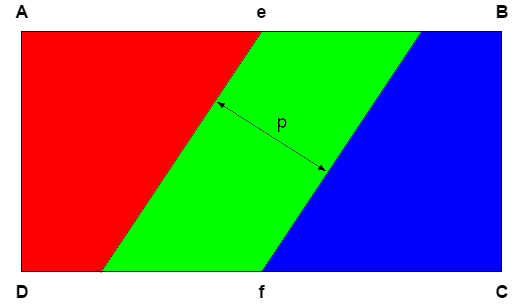# Find The Area

Geometry Level 2The rectangle $ABCD$ is twice as wide as it is high. Parallel lines which are a distance of $p$ apart cross the mid-point of the long sides of the rectangle at $e$ and $f$ as shown. All 3 regions (red, green, blue) are of equal area.

What is the area of the rectangle $ABCD$ as a function of $p$?

×

Problem Loading...

Note Loading...

Set Loading...